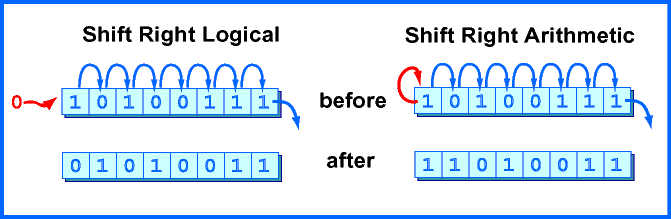### Answer:

```1111 1111 1111 0000  →  0011 1111 1111 1100
```

Is the resulting pattern the correct representation for -16/4? No. The result represents a large positive number, not -4

# Shift Right Arithmetic

A right shift logical can not be used to divide a negative integer by two. The problem is that a shift right logical moves zeros into the high order bit. This is desirable in some situations, but not for dividing negative integers where the high order bit is the "sign bit." An arithmetic right shift replicates the sign bit as needed to fill bit positions:### QUESTION 13:

Is there a need for an arithmetic shift left instruction?